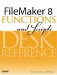# Log()

Category: Number

 Syntax: Log ( number )

Parameters:

numberAny positive number or expression that returns a positive number.

Data type returned: Number

Description:

Returns the base-10 logarithm of number.

Logarithms are used to determined the power to which a number must be raised to equal some other number. If xn = y, then n = Logx(y). The Log() function assumes a base (the variable x in the preceding formula) of 10. The Lg() function uses a base of 2, whereas the Ln() function uses a base of e.

The Log() and Lg() functions produce base-10 and base-2 logarithms, respectively. The Ln() function produces base-e logarithms, but it can also be used to solve a logarithm of any base. Log (base-x) of y is equivalent to Ln (y) / Ln (x).

Examples:

Function

Results

Log (1)

Returns 0 because 10^0 = 1.

Log (100)

Returns 2 because 10^2 = 100.

Log (1000)

Returns 3 because 10^3 = 1000.FileMaker 8 Functions and Scripts Desk Reference
ISBN: 0789735113
EAN: 2147483647
Year: 2004
Pages: 352